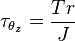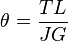# Torsion (mechanics) facts for kids

Kids Encyclopedia Facts

In solid mechanics, torsion is the twisting of an object that is result of an applied torque. In circular sections, the resultant shearing stress is perpendicular to the radius.

The shear stress at a point on a shaft is:$\tau_{\theta_{z}} = {T r \over J}$

T is the applied torque, r is the distance from the center of rotation, and J is the polar moment of inertia.

The angle of twist can be found by using:$\theta_{} = {T L \over JG}$

Where:

• θ is the angle of twist in radians.
• T is the torque (N·m or ft·lbf).
• L is the length of the object the torque is being applied to or over.
• G is the shear modulus or more commonly the modulus of rigidity and is usually given in gigapascals (GPa) or ft·lbf.
• J is the polar moment of inertia, for a round shaft or concentric tube only. For other shapes J must be determined by other means. For solid shafts the membrane analogy is useful, and for thin walled tubes of arbitrary shape the shear flow approximation is fairly good, if the section is not re-entrant. For thick walled tubes of arbitrary shape there is no simple solution, FEA may be the best method.

## Images for kidsTorsion (mechanics) Facts for Kids. Kiddle Encyclopedia.